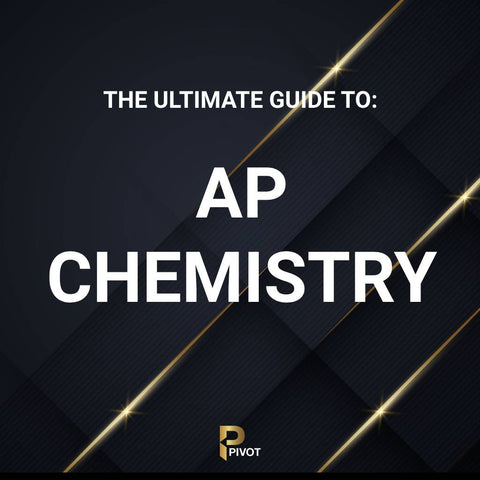# How the AP Chemisty Exam is Scored

The AP Chemistry exam's scoring can initially seem complex, but understanding the breakdown can provide clarity and even offer strategic insights for test-takers. Here's how the point system works:

• Multiple Choice Section:
• Weightage: This section typically constitutes about 50% of your total score.
• Questions: It usually consists of around 60 questions.
• Scoring: Each question is worth one point, and there's no penalty for incorrect answers. Thus, it's to your advantage to answer every question, even if you're unsure.
• Free Response Section:
• Weightage: This section also makes up approximately 50% of your total score.
• Components: It's generally divided into three long essay questions and four short-answer questions.
• Scoring Breakdown: Each long essay question can usually earn a student up to 10 points, while short answers typically have a maximum of 4 points each. The exact point allocation can vary based on the complexity and components of each question.
• Details: Points are awarded for specific parts of each answer. For instance, in a calculation question, points might be distributed for correct setup, the calculation itself, and the correct answer. In theory-based questions, points might be given for each correct concept or explanation provided.
• Composite Score to AP Score Conversion:
• After your points from both sections are tallied, they're converted into a composite score. This score is then translated into the AP's 5-point scale:
• 5: Extremely well qualified
• 4: Well qualified
• 3: Qualified
• 2: Possibly qualified
• 1: No recommendation

Ultimately, a holistic understanding of the AP Chemistry exam's structure and scoring system, combined with thorough preparation, sets the stage for achieving a top score.